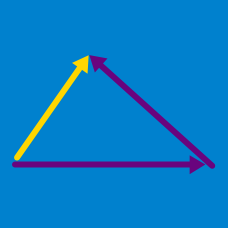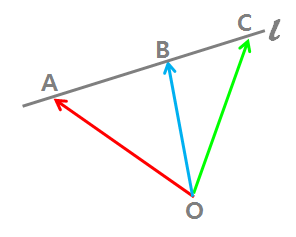Geometry

Vector Terminology

Which of the following is a vector quantity?

1. The displacement from New York to London
2. The color of a shoe
3. The number of books on a shelf
4. An array with the number of books reads by each student in a class
5. The speed of an airplane

Which of the following vectors in the regular hexagon below has the same magnitude as $\vec{AB}$ but opposite direction?Details and assumptions:

• O is the intersection point of the three diagonal lines.

If $\vec{a}=\vec{b},$ which of the following is/are true?

I. $\vec{a}$ and $\vec{b}$ are parallel.
II. $\vec{a}$ and $\vec{b}$ are equal in magnitude.
III. The initial points of $\vec{a}$ and $\vec{b}$ are the same.
IV. The terminal points of $\vec{a}$ and $\vec{b}$ are the same.

Let $\vec{OA}=\vec{a}+2\vec{b}, \vec{OB}=3\vec{a}-\vec{b},$ and $\vec{OC}=2\vec{a}+k\vec{b}$ in the diagram below. If the three points $A, B,$ and $C$ lie on the line $l$, which of the following is equal to $k$?Details and assumptions:

• Point $O$ does $\color{#D61F06}{\text{not}}$ lie on the line $l.$

Find the unit vector in the direction $(1,2,3).$

×